# PSEB 7th Class Maths Solutions Chapter 2 Fractions and Decimals Ex 2.1

Punjab State Board PSEB 7th Class Maths Book Solutions Chapter 2 Fractions and Decimals Ex 2.1 Textbook Exercise Questions and Answers.

## PSEB Solutions for Class 7 Maths Chapter 2 Fractions and Decimals Ex 2.1

1. Solve the following fractions :

Question (i).
4 + $$\frac {7}{8}$$
4 + $$\frac {7}{8}$$
= $$\frac{4 \times 8+7}{8}$$
= $$\frac{32+7}{8}$$
= $$\frac {39}{8}$$
= 4$$\frac {7}{8}$$

Question (ii).
$$\frac{9}{11}-\frac{4}{15}$$
$$\frac{9}{11}-\frac{4}{15}$$
= $$\frac{9 \times 15-4 \times 11}{11 \times 15}$$
= $$\frac{135-44}{165}$$
= $$\frac {91}{165}$$

Question (iii).
$$\frac{11}{16}-\frac{2}{5}+\frac{8}{10}$$
$$\frac{11}{16}-\frac{2}{5}+\frac{8}{10}$$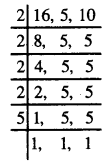LCM of 16, 5 and 10
= 2 × 2 × 2 × 2 × 5
= 80
= $$\frac{11 \times 5-2 \times 16+8 \times 8}{80}$$
= $$\frac{55-32+64}{80}$$
= $$\frac {87}{80}$$
= 1$$\frac {7}{80}$$

Question (iv).
$$2 \frac{1}{5}+6 \frac{1}{2}$$
$$2 \frac{1}{5}+6 \frac{1}{2}$$
= $$\frac{11}{5}+\frac{13}{2}$$
= $$\frac{11 \times 2+13 \times 5}{5 \times 2}$$
= $$\frac{22+65}{10}$$
= $$\frac {87}{10}$$
= 8$$\frac {7}{10}$$

Question (v).
$$8 \frac{1}{2}-3 \frac{5}{8}$$
$$8 \frac{1}{2}-3 \frac{5}{8}$$
= $$\frac{17}{2}-\frac{29}{8}$$
= $$\frac{17 \times 4-29}{8}$$
= $$\frac{68-29}{8}$$
= $$\frac {39}{8}$$
= 4$$\frac {7}{8}$$Question (vi).
$$\frac{9}{10}-\frac{9}{100}+\frac{9}{1000}$$
$$\frac{9}{10}-\frac{9}{100}+\frac{9}{1000}$$
= $$\frac{9 \times 100-9 \times 10+9}{1000}$$
= $$\frac{900-90+9}{1000}$$
= $$\frac {810}{1000}$$

2. Arrange the following in ascending order :

Question (i).
$$\frac{2}{17}, \frac{10}{17}, \frac{3}{17}, \frac{16}{17}, \frac{5}{17}, \frac{8}{17}$$
Ascending order of $$\frac{2}{17}, \frac{10}{17}, \frac{3}{17}, \frac{16}{17}, \frac{5}{17}, \frac{8}{17}$$ is:
$$\frac{2}{17}, \frac{3}{17}, \frac{5}{17}, \frac{8}{17}, \frac{10}{17}, \frac{16}{17}$$

Question (ii).
$$\frac{1}{5}, \frac{3}{7}, \frac{7}{10}$$
$$\frac{1}{5}, \frac{3}{7}, \frac{7}{10}$$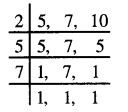L.C.M of 5, 7 , 10 = 2 × 5 × 7
= 70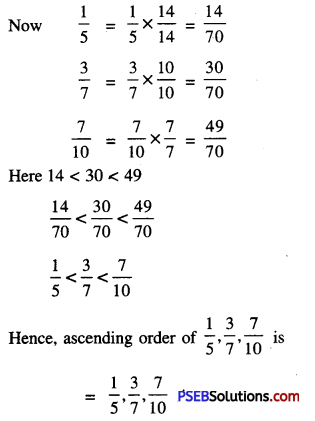3. The three sides AB, BC and CA of a triangle ΔABC are $$\frac {5}{6}$$cm, $$\frac {2}{3}$$cm and $$\frac {7}{10}$$ cm respectively. Find the perimeter of the triangle.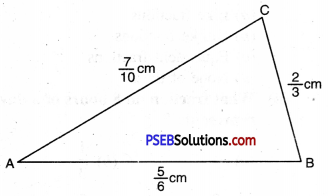Slides of ΔABC are
AB = $$\frac {5}{6}$$ cm,
BC = $$\frac {2}{3}$$
CA = $$\frac {7}{10}$$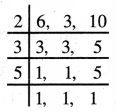L.C.M. (6, 3, 10) = 2 × 3 × 5 = 30
Perimeter of ΔABC = AB + BC + CA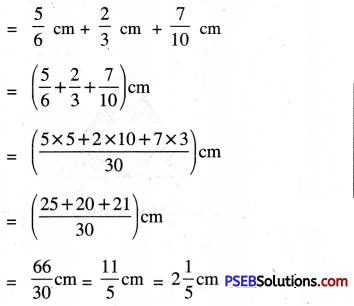4. Ramesh studies for 5$$\frac {2}{3}$$ hours daily. He devotes 2$$\frac {4}{5}$$ hours of his time for science devotes for other subjects ?
Total daily time for all subjects
= 5$$\frac {2}{3}$$ hours = $$\frac {17}{3}$$ hours
Time for science and mathematics
= 2$$\frac {4}{5}$$ hours = $$\frac {14}{5}$$ hours
Time for other subjects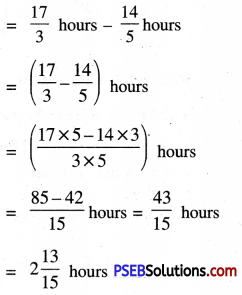5. Sonia jogs once around the rectangular park of sides 10$$\frac {2}{3}$$m and 12$$\frac {1}{2}$$m. Find the total distance covered by the Sonia.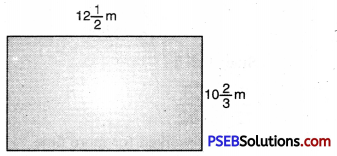Length of rectangular park
= 12$$\frac {1}{2}$$m = $$\frac {25}{2}$$m
= 10$$\frac {2}{3}$$m = $$\frac {32}{3}$$m
Total distance covered by Sonia = 2 [Length + Breadth]
= $$2\left(\frac{32}{3}+\frac{25}{3}\right) \mathrm{m}$$
= $$2\left(\frac{32 \times 2+25 \times 3}{3 \times 2}\right) \mathrm{m}$$
= $$2\left(\frac{65+75}{6}\right) \mathrm{m}$$
= $$\frac {278}{6}$$ m
= $$\frac {139}{3}$$ m
= 46$$\frac {1}{3}$$ m

6. Ritu coloured a picture in $$\frac {7}{12}$$ hours. Vaibhav coloured the same picture in $$\frac {3}{4}$$ hours. Who worked for a longer time and by what fraction ?
Time taken by Ritu to colour
= $$\frac {7}{12}$$ hours
Time taken by Vaibhav = $$\frac {3}{4}$$ hours
= $$\frac {3}{4}$$ × $$\frac {3}{3}$$
= $$\frac {9}{12}$$ hours
Since 9 > 7
∴ $$\frac {9}{12}$$ > $$\frac {7}{12}$$
∴ Vaibhav worked for more time.
Difference between time taken by
Vaibhav and Ritu = $$\frac{3}{4}-\frac{7}{12}$$
= $$\frac{3 \times 3-7}{12}$$
= $$\frac{9-7}{12}=\frac{2}{12}$$
= $$\frac {1}{6}$$ of an hour.7. Multiple Choice Questions :

Question (i).
Fraction $$\frac {2}{5}$$, $$\frac {7}{5}$$ are :
(a) Like fractions
(b) Unlike fractions
(c) Equivalent fractions
(d) None of these
(a) Like fractions

Question (ii).
What fraction do 8 hours of a day represents ?
(a) $$\frac {1}{2}$$
(b) $$\frac {1}{3}$$
(c) $$\frac {8}{60}$$
(d) $$\frac {2}{3}$$
(b) $$\frac {1}{3}$$

Question (iii).
Equivalent fraction of $$\frac {3}{5}$$ is :
(a) $$\frac {13}{15}$$
(b) $$\frac {5}{3}$$
(c) $$\frac {9}{15}$$
(d) $$\frac {5}{13}$$
(c) $$\frac {9}{15}$$

Question (iv).
Shaded area of given triangle represents the fractions:
(a) $$\frac {1}{3}$$
(b) $$\frac {3}{4}$$
(c) $$\frac {1}{4}$$
(d) $$\frac {2}{3}$$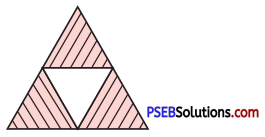(b) $$\frac {3}{4}$$
Sum of fractions $$\frac {2}{7}$$, $$\frac {3}{4}$$ is equal to :
(a) $$\frac {5}{28}$$
(b) $$\frac {1}{3}$$
(c) $$\frac {5}{11}$$
(d) $$\frac {29}{28}$$
(d) $$\frac {29}{28}$$